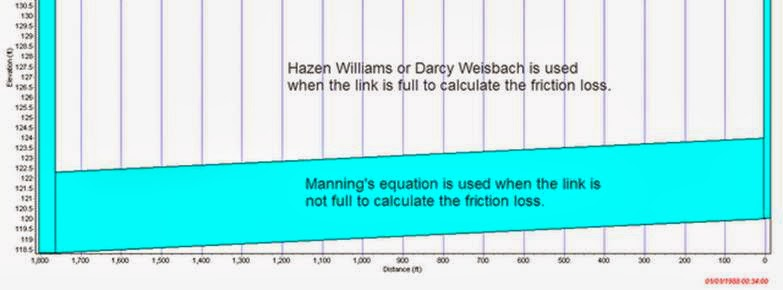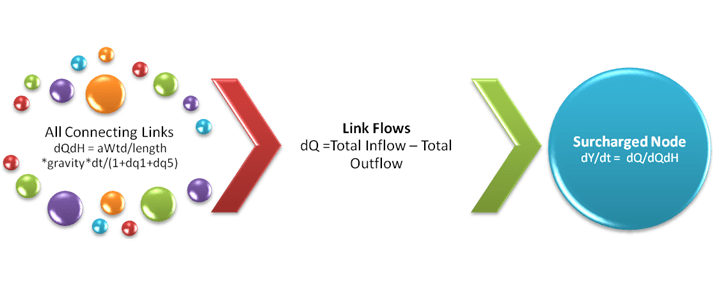#INFOSWMM

# Force Main Friction Loss in InfoSWMM and the Transition from Partial to Full Flow

Subject:  Force Main Friction Loss in InfoSWMM and the Transition from Partial to Full Flow

You can model Force Main friction loss in InfoSWMM using either Darcy Weisbach or Hazen Williams as the full pipe friction loss method (see Figure 1 for the internal definition of full flow).   A function called ForceMain in InfoSWMM whose purpose is to compute the Darcy-Weisbach friction factor for a force main using the Swamee and Jain approximation to the Colebrook-White equation No matter which method you use for full flow the  program will use Manning's equation to calculate the loss in the link when the link is not full (see Figure 2 for the equations used for calculating the friction loss – variable dq1 in the St Venant equation for InfoSWMM).   The regions for the different friction loss equations are shown in Figure 3.
There is no slot in InfoSWMM for the full pipe flow as a surcharged node in InfoSWMM uses this point iteration equation (Figure 4):
dY/dt = dQ / The sum of the Connecting Link values of  dQ/dH
where Y is the depth in the node, dt is the time step, H is the head across the link (downstream – upstream), dQ is the net inflow into the node and dQ/dH is the derivative with respect to H of the link  St Venant equation.  If you are trying to calibrate the surcharged node depth, the main calibration variables are the time step and the link  roughness:
1.   Mannings's N
2.   Hazen-Williams or
3.   Darcy-Weisbach
The link roughness is part of the term dq1 in the St Venant solution and the other loss terms are included in the term dq5.  You can adjust the roughness of the surcharged link  to affect the node surcharge depth.   The point iteration continues until the sum of the flow in the node is zero – basically the new depth in the node either increases or decreases the friction loss in the force main so that net flow at the node is zero.  This is why it is important to use the right time step to ensure that the net flow is zero when the pumps turn on and off.
Figure 1.  How the full pipe condition is defined in InfoSWMM - both ends have to be full
Figure 2:  Friction equations used in SWMM 5 for a Force Main.Figure 3:  Regions of Friction loss equations in SWMM 5.

Figure 4.  The Node Surcharge Equation is a function of the net inflow and the sum of the term dQ/dH in all connecting links. Generally, as you increase the roughness the value of dQ/dH increases and the denominator of the term dY/dt = dQ/dQdH increases.